# Three-Digit Subtraction with Counting Strategies: Grade 2

##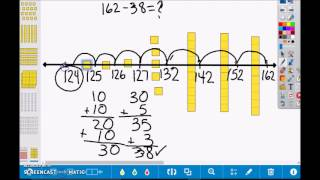By university of Houston mathematics education

The video describes counting strategies using place value to subtract.# [3.NBT.2-2.0] Fluent Add/Sub - Common Core Standard - Word Problem

##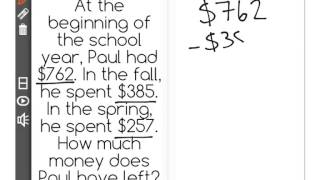By Freckle education

Fluently subtract within 1000 using strategies and algorithms based on place value, properties of operations, and/or the relationship between addition and subtraction.# Subtraction

##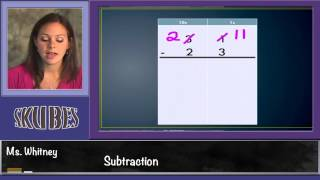By Skubes ed

Fluently add and subtract within 1000 using strategies and algorithms based on place value, properties of operations, and/or the relationship between addition and subtraction.# eSparkLearning: Adding & Subtracting within 1000 Framing Video (3NBT, Quest 3)

##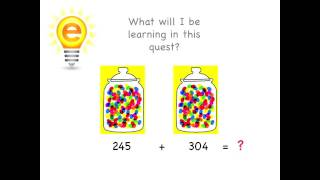By espark learning videos

Adding & Subtracting within 1000 using strategies and algorithms based on place value, properties of operations# Three digit-two digit subtraction with regrouping:grade 2

##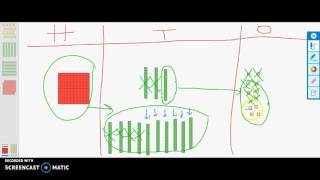By university of Houston mathematics education

The video describes a student strategy for subtraction with regrouping using concrete, pictorial and abstract models.# [3.NBT.2-1.0] Fluent Add/Sub - Common Core Standard

##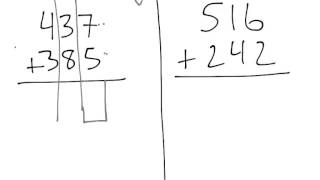By Freckle education

Fluently add within 1000 using strategies and algorithms based on place value, properties of operations, and/or the relationship between addition and subtraction.# Three digit-two digit subtraction with regrouping tens:grade 2

##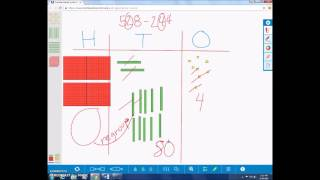By university of Houston mathematics education

The video describes student strategies for three digit subtraction regrouping tens using concrete, pictorial and abstract models.# Vectors : scalar multiplication, addition and subtraction : ExamSolutions

##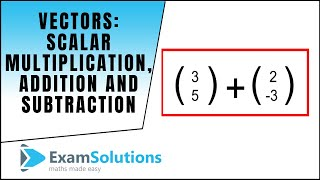By ExamSolutions

Youtube Presents Vectors : scalar multiplication, addition and subtraction : ExamSolutions an educational video resources on english language arts.# Subtracting 1 vs. subtracting 10 | Addition and subtraction within 100

##By Khan Academy

Sal talks about the difference between subtracting 1 and subtracting 10.# Addition and subtraction trig identities

##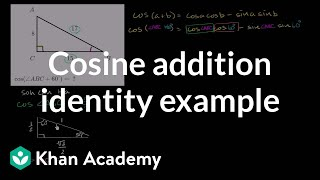By Khan Academy

Sal evaluates the cosine of the sum of 60ï¿½ï¿½ï¿½ï¿½ï¿½ï¿½ï¿½ï¿½ï¿½ï¿½ï¿½ï¿½ and another angle whose right triangle is given. To do this, he must use the cosine angle addition formula.# More examples of addition and subtraction of polynomials | Algebra II | Khan Academy

##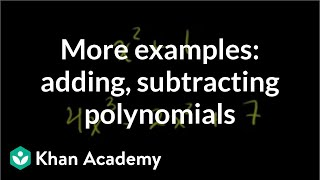By Khan Academy

This instructor in this video, Sal Khan, discusses adding and subtracting polynomials. In an easy, conversational tone, the instructor explains processes clearly. Mr. Khan uses the Paint Program (with different colors) to illustrate his points. Sal Khan is the recipient of the 2009 Microsoft Tech Award in Education. The student or educator may want to open the video to 'full screen' as the instructor is using a black background and the writing is small.# Addition and subtraction with number lines

##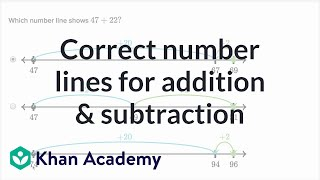By Khan Academy

Sal figures out which number line matches up with a 2-digit addition or subtraction problem.# Addition and subtraction trig identities

##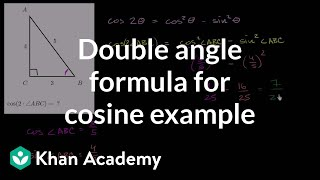By Khan Academy

Sal evaluates the cosine of twice anï¿½ï¿½ï¿½ï¿½ï¿½ï¿½ï¿½ï¿½ï¿½ï¿½ï¿½ï¿½angle whose right triangle is given. To do this, he must use the cosine double-angle formula.##By Khan Academy

Sal solves missing number in problems like 73 = ___ + 57.##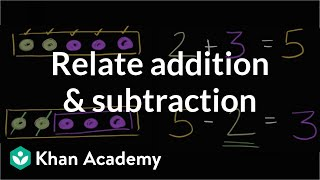By Khan Academy

See the relationship between 2+3=5, 5-2=3, and 5-3=2.# Addition and subtraction trig identities

##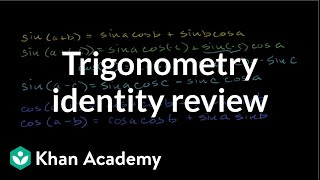By Khan Academy

Sal reviews all the different trigonometric angle addition identities: sin(a+b), sin(a-c), cos(a+b), cos(a-b), cos(2a), and sin(2a).# [4.NF.3a-1.0] Intro to Fraction Addition - Common Core Standard - Word Problem

##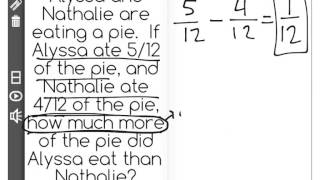By Freckle education

Understand a fraction a/b with a greater than 1 as a sum of fractions 1/b. Understand addition and subtraction of fractions as joining and separating parts referring to the same whole.# [4.NF.3a-1.0] Intro to Fraction Addition - Common Core Standard

##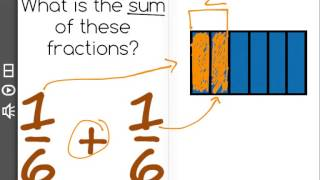By Freckle education

Understand a fraction a/b with a greater than 1 as a sum of fractions 1/b. Understand addition and subtraction of fractions as joining and separating parts referring to the same whole.##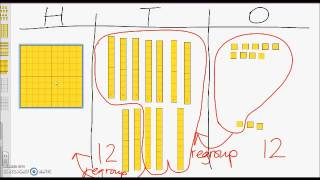By university of Houston mathematics education

The video describes how elementary students can solve an addition word problem regrouping tens and ones.# Addition & Subtraction of Mixed Numbers: With Same Denominators

##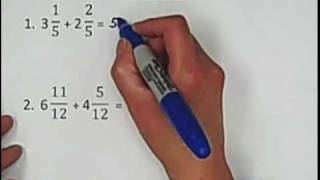By Red River College -Tutoring

Addition & Subtraction of Mixed Numbers: With Same Denominators